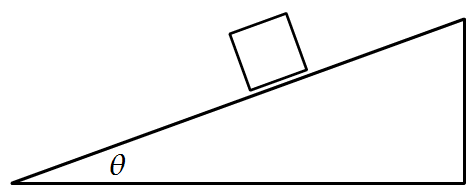# Inclined PlaneA cubical block of mass $m$ is released from rest at a height $h$ on a frictionless surface of a movable wedge of mass $M$, which in turn is placed on a horizontal frictionless surface as shown in the figure.

The magnitude of velocity of the triangular block when the smaller block reaches the bottom is

$\large v = { \left [ \dfrac{a m^b g h \cos ^{ c }{ \theta } }{( m + M)( M + m\sin ^{ d }{ \theta } )} \right]}^{ e }$

Enter your answer as $a + b + c + d + e$.

$Details$ $and$ $Assumptions$:

• $a$,$b$,$c$,$d$ are positive integers and $e$ is a positive rational number

• Velocity is asked when the block reaches the bottom of the incline ( not the horizontal surface).

• All surfaces of contacts are frictionless.

• Size of block is negligible compared to size of the incline.

×